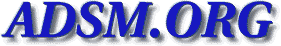Networker [Top] [All Lists]

## [Networker] Session statistics broken for over 2Tb.

2012-10-25 11:48:31
 Subject: [Networker] Session statistics broken for over 2Tb. Yaron Zabary NETWORKER AT LISTSERV.TEMPLE DOT EDU Thu, 25 Oct 2012 17:48:21 +0200
 ```Hello all, ``` I have this script which tries to dig some statistics from nsradmin's session statistics. It works nicely for sessions smaller than 2Tb, but breaks above that. I suspect that nsradmin does 32 bit counters. For example: ``` [root@legato ~]# nsradmin NetWorker administration program. Use the "help" command for help, "visual" for full-screen mode. nsradmin> . type: NSR Current query set nsradmin> option hidden; Hidden display option turned on Display options: Dynamic: Off; Hidden: On; Raw I18N: Off; Resource ID: Off; Regexp: Off; nsradmin> option dynamic Dynamic display option turned on Display options: Dynamic: On; Hidden: On; Raw I18N: Off; Resource ID: Off; Regexp: Off; nsradmin> show session statistics nsradmin> print session statistics: id = 285113144, jobid = 0, name = dayan-ng.tau.ac.il, mode = browsing, ``` "group = ", "pool = ", "volume = ", rate kb = 0, amount kb = 0, total kb = 0, amount files = 0, ``` total files = 0, start time = 1350993680, connect time = 185605, num volumes = 0, used volumes = 0, completion = running, flags = 0, "level = ", id = 285113524, jobid = 76501, name = cloning session, ``` mode = recovering, "group = ", pool = DDPool, ``` volume = DDPool.001.RO, rate kb = 0, ``` amount kb = 129176321, total kb = 1018277321, ``` amount files = 0, total files = 0, ``` start time = 1351029303, connect time = 149982, ``` num volumes = 0, used volumes = 0, completion = running, flags = 4, "level = ", id = 285113525, jobid = 76501, name = legato.tau.ac.il, mode = saving, ``` "group = ", pool = TAUDefault, volume = JDF648, ``` rate kb = 0, amount kb = 0, total kb = 0, amount files = 0, total files = 0, ``` start time = 1351029303, connect time = 149982, ``` num volumes = 0, used volumes = 5, completion = running, flags = 26, "level = "; nsradmin> [root@legato ~]# /usr/local/TAUSRC/Local/ToolBox/monstage.pl 76501 r=0MB/s size=841GB/971GB time=16205m ETA=5/23:43 The size is reported correctly with nsradmin's session attribute: [root@legato ~]# /usr/local/TAUSRC/Local/ToolBox/showsessions.pl|nl 1 dayan-ng.tau.ac.il:root browsing ``` 2 cloning session:1 of 7 save set(s) reading from DDPool.001.RO 4431 GB of 5313 GB 3 legato.tau.ac.il:cloning session saving to pool 'TAUDefault' (JDF648) ``` ``` NMC is no better. It thinks that the size of this staging session is 1018Gb. I had this investigated under SR#44358972, but they claimed that this was OK with 7.6.3HF and was related to NW138153. Networker is now 7.6.4.2.Build.1060, but the problem is still here. ``` Does anyone knows which version has this corrected ? #!/usr/bin/perl use lib "/usr/local/TAUSRC/Local/ToolBox"; use Nsradmin; require "timelocal.pl"; set_nsradmin("/usr/sbin/nsradmin"); \$server = "legato"; \$query = "type: NSR "; \$show = "session statistics"; \$options = "hidden; dynamic"; @reslist = query(\$server, \$query, \$show, \$options); # # A reslist is a list of resources. Resources are a # hash of attributes, which have a name and value lists. # \$found = 0; foreach \$res (@reslist) { %attrlist = %{\$res}; \$attr = "session statistics"; @vallist = @{\$attrlist{\$attr}}; foreach \$val (@vallist) { if (\$val =~ "jobid") { (\$a,\$jobid) = split(/ = /,\$val); } if (\$val =~ "total kb") { (\$a,\$totalkb) = split(/ = /,\$val); } if (\$val =~ "amount kb") { (\$a,\$amountkb) = split(/ = /,\$val); } if (\$val =~ "connect time") { (\$a,\$ctime) = split(/ = /,\$val); \$rate = \$amountkb/\$ctime; if (\$found == 1) { #print "\$totalkb \$amountkb \n"; \$left = \$totalkb - \$amountkb; \$leftt = \$left/\$rate if \$rate > 0; \$eta = time() + \$leftt; ``` (\$sec,\$min,\$hour,\$mday,\$monx,\$year,\$wday,\$yday,\$isdst) = localtime(\$ ```eta); \$rate = int(\$rate/1024); \$left = int(\$left/1024/1024); \$leftt = int(\$leftt/60); \$totalkb = int(\$totalkb/1024/1024); printf "%d r=%dMB/s size=%dGB/%dGB time=%dm ETA=%d/%d:%02d\n", ``` \$jobid,\$rate,\$left,\$totalkb,\$leftt,\$mday,\$hour,\$min if (\$found ``` == 1); \$found = 0; #last; } } \$found = 1 if (\$val =~ 'cloning session'); } } -- -- Yaron. ```
 Current Thread [Networker] Session statistics broken for over 2Tb., Yaron Zabary <= Re: [Networker] Session statistics broken for over 2Tb., Tony Albers Re: [Networker] Session statistics broken for over 2Tb., Yaron Zabary Re: [Networker] Session statistics broken for over 2Tb., Francis Swasey Re: [Networker] Session statistics broken for over 2Tb., Yaron Zabary Re: [Networker] Session statistics broken for over 2Tb., Francis Swasey Re: [Networker] Session statistics broken for over 2Tb., Yaron Zabary Re: [Networker] Session statistics broken for over 2Tb., Francis Swasey